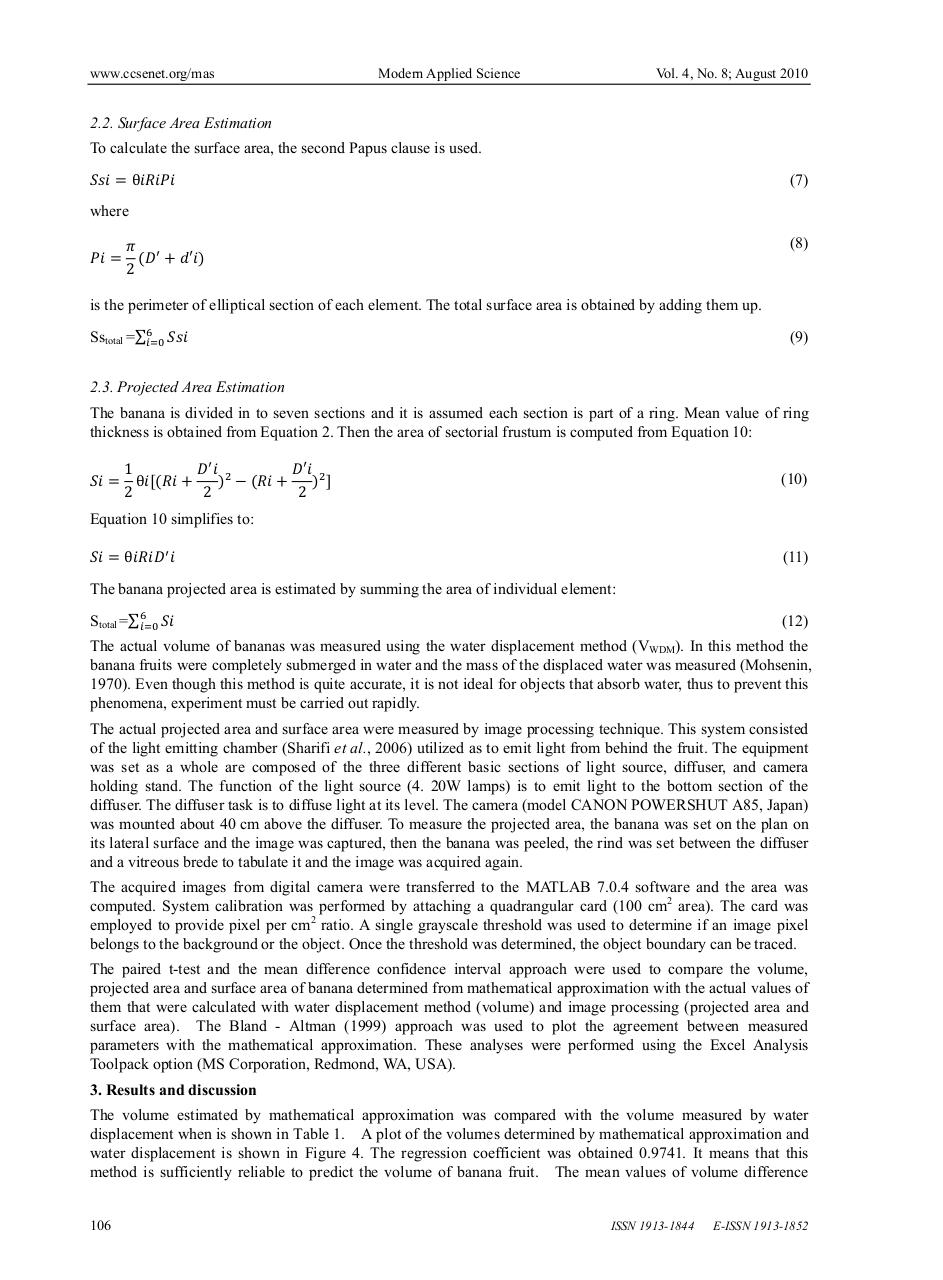# 5528.pdfPage 1 2 3 4 5 6 7 8 9 10

#### Text preview

www.ccsenet.org/mas

Modern Applied Science

Vol. 4, No. 8; August 2010

2.2. Surface Area Estimation
To calculate the surface area, the second Papus clause is used.
θ

(7)

where
(8)
2
is the perimeter of elliptical section of each element. The total surface area is obtained by adding them up.
Sstotal =∑

(9)

2.3. Projected Area Estimation
The banana is divided in to seven sections and it is assumed each section is part of a ring. Mean value of ring
thickness is obtained from Equation 2. Then the area of sectorial frustum is computed from Equation 10:
1
θ
2

2

(10)

2

Equation 10 simplifies to:
θ

(11)

The banana projected area is estimated by summing the area of individual element:
Stotal =∑

(12)

The actual volume of bananas was measured using the water displacement method (VWDM). In this method the
banana fruits were completely submerged in water and the mass of the displaced water was measured (Mohsenin,
1970). Even though this method is quite accurate, it is not ideal for objects that absorb water, thus to prevent this
phenomena, experiment must be carried out rapidly.
The actual projected area and surface area were measured by image processing technique. This system consisted
of the light emitting chamber (Sharifi et al., 2006) utilized as to emit light from behind the fruit. The equipment
was set as a whole are composed of the three different basic sections of light source, diffuser, and camera
holding stand. The function of the light source (4. 20W lamps) is to emit light to the bottom section of the
diffuser. The diffuser task is to diffuse light at its level. The camera (model CANON POWERSHUT A85, Japan)
was mounted about 40 cm above the diffuser. To measure the projected area, the banana was set on the plan on
its lateral surface and the image was captured, then the banana was peeled, the rind was set between the diffuser
and a vitreous brede to tabulate it and the image was acquired again.
The acquired images from digital camera were transferred to the MATLAB 7.0.4 software and the area was
computed. System calibration was performed by attaching a quadrangular card (100 cm2 area). The card was
employed to provide pixel per cm2 ratio. A single grayscale threshold was used to determine if an image pixel
belongs to the background or the object. Once the threshold was determined, the object boundary can be traced.
The paired t-test and the mean difference confidence interval approach were used to compare the volume,
projected area and surface area of banana determined from mathematical approximation with the actual values of
them that were calculated with water displacement method (volume) and image processing (projected area and
surface area). The Bland - Altman (1999) approach was used to plot the agreement between measured
parameters with the mathematical approximation. These analyses were performed using the Excel Analysis
Toolpack option (MS Corporation, Redmond, WA, USA).
3. Results and discussion
The volume estimated by mathematical approximation was compared with the volume measured by water
displacement when is shown in Table 1. A plot of the volumes determined by mathematical approximation and
water displacement is shown in Figure 4. The regression coefficient was obtained 0.9741. It means that this
method is sufficiently reliable to predict the volume of banana fruit. The mean values of volume difference
106

ISSN 1913-1844

E-ISSN 1913-1852